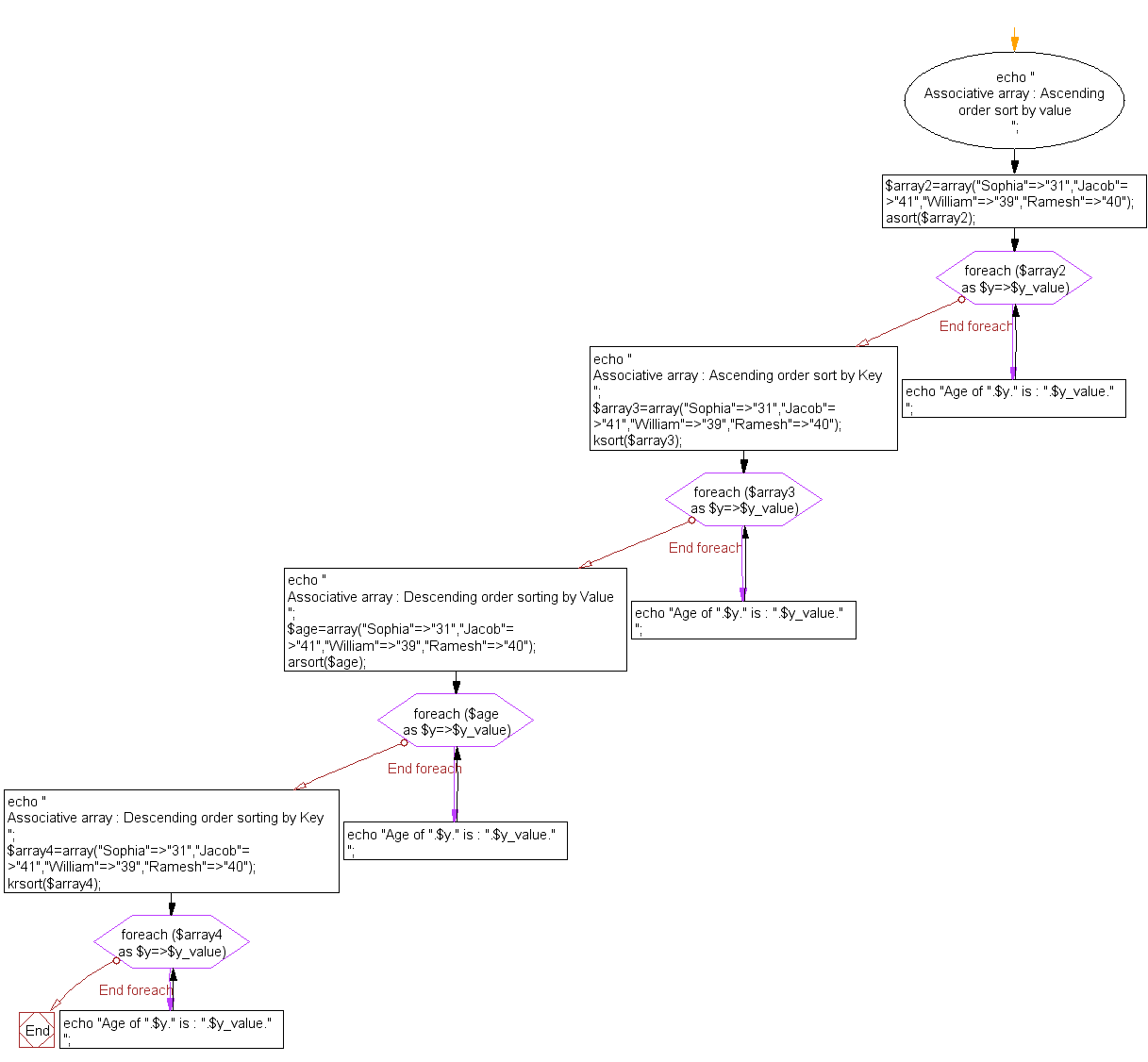﻿ PHP Array Exercise: Sort an associative array - w3resource# PHP Array Exercises : Sort an associative array

## PHP Array: Exercise-8 with Solution

Write a PHP script to sort the following associative array :

array("Sophia"=>"31","Jacob"=>"41","William"=>"39","Ramesh"=>"40") in
a) ascending order sort by value
b) ascending order sort by Key
c) descending order sorting by Value
d) descending order sorting by Key

Sample Solution:

PHP Code:

``````<?php
echo "
Associative array : Ascending order sort by value
";
\$array2=array("Sophia"=>"31","Jacob"=>"41","William"=>"39","Ramesh"=>"40"); asort(\$array2);
foreach(\$array2 as \$y=>\$y_value)
{
echo "Age of ".\$y." is : ".\$y_value."
";
}
echo "
Associative array : Ascending order sort by Key
";
\$array3=array("Sophia"=>"31","Jacob"=>"41","William"=>"39","Ramesh"=>"40"); ksort(\$array3);
foreach(\$array3 as \$y=>\$y_value)
{
echo "Age of ".\$y." is : ".\$y_value."
";
}
echo "
Associative array : Descending order sorting by Value
";
\$age=array("Sophia"=>"31","Jacob"=>"41","William"=>"39","Ramesh"=>"40");
arsort(\$age);
foreach(\$age as \$y=>\$y_value)
{
echo "Age of ".\$y." is : ".\$y_value."
";
}
echo "
Associative array : Descending order sorting by Key
";
\$array4=array("Sophia"=>"31","Jacob"=>"41","William"=>"39","Ramesh"=>"40"); krsort(\$array4);
foreach(\$array4 as \$y=>\$y_value)
{
echo "Age of ".\$y." is : ".\$y_value."
";
}
?>
```
```

Sample Output:

```Associative array : Ascending order sort by value
Age of Sophia is : 31
Age of William is : 39
Age of Ramesh is : 40
Age of Jacob is : 41

Associative array : Ascending order sort by Key
Age of Jacob is : 41
Age of Ramesh is : 40
Age of Sophia is : 31
Age of William is : 39

Associative array : Descending order sorting by Value
Age of Jacob is : 41
Age of Ramesh is : 40
Age of William is : 39
Age of Sophia is : 31

Associative array : Descending order sorting by Key
Age of William is : 39
Age of Sophia is : 31
Age of Ramesh is : 40
Age of Jacob is : 41
```

Flowchart:PHP Code Editor:

What is the difficulty level of this exercise?

Test your Programming skills with w3resource's quiz.

﻿

## PHP: Tips of the Day

Shorthand notation for echo: When outside of PHP tags, a shorthand notation for echo is available by default, using to end it.

Example:

```<?=\$variable?>
<?= "This is PHP Tips" ?>
```

Output:

```This is PHP Tips
```

Note that there is no terminating ;. This works because the closing PHP tag acts as the terminator for the single statement. So, it is conventional to omit the semicolon in this shorthand notation.

Priority of print

Although the print is language construction it has priority like operator. It places between = += -= *= **= /= .= %= &= and and operators and has left association.

Example:

```<?php
echo '2' . print '5' + 3;
?>
```

Output:

```821
```

Same example with brackets:

```<?php
echo '2' . print ('5' + 3);
?>
```

Output:

```821
```